﻿ Design of Nonlinear CSTR Control System using Active Disturbance Rejection Control Optimized by Asexual Reproduction Optimization

### Design of Nonlinear CSTR Control System using Active Disturbance Rejection Control Optimized by Asex...

Navid Yazdanparast, Mehdi Shahbazian, Masoud Aghajani, Saeed Pour AbedOPEN ACCESSPEER-REVIEWED

## Design of Nonlinear CSTR Control System using Active Disturbance Rejection Control Optimized by Asexual Reproduction Optimization

Navid Yazdanparast1,, Mehdi Shahbazian1, Masoud Aghajani2, Saeed Pour Abed3

1Department of Instrumentation and Automation Engineering, Petroleum University of Technology, Ahwaz, Iran

2Department of Chemical Engineering, Petroleum University of Technology, Ahwaz, Iran

3Senior expert in Instrumentation Unit, Sarkhoon&Qeshm Gas treating Company, Bandar Abbas, Iran

### Abstract

This paper proposes an optimal Active Disturbance Rejection Control (ADRC) based on using Asexual Reproduction Optimization (ARO) to control the temperature of a nonlinear CSTR. The parameters of non-isothermal continuous stirred tank reactor (CSTR) are varying with time caused by fouling and the deactivation and regeneration of the catalyst. Furthermore, in the exothermal region, dynamic behavior of this reactor is unstable. Therefore, designing an efficient controller in this complicated situation is difficult and challenging. ADRC is used as a robust method to control the temperature of CSTR in the situation that the CSTR parameters are varying with time. The parameters of ADRC are difficult to adjust and if these parameters tuned properly, it performs more efficiently in setpoint tracking and disturbance rejection. In this paper Controller design is represented as an optimization problem. The parameters of ADRC are tuned by ARO and then by Particle Swarm Optimization (PSO). The performance of ADRC tuned by ARO (ADRC-ARO) is compared with the performance of ADRC tuned by PSO (ADRC-PSO) and PID controller. The simulation results that the proposed ADRC-ARO method reveals robustness and better performance in both setpoint tracking and disturbance rejection with faster response time and less settling time.

### At a glance: Figures

12345
Prev Next

• Yazdanparast, Navid, et al. "Design of Nonlinear CSTR Control System using Active Disturbance Rejection Control Optimized by Asexual Reproduction Optimization." Journal of Automation and Control 3.2 (2015): 36-42.
• Yazdanparast, N. , Shahbazian, M. , Aghajani, M. , & Abed, S. P. (2015). Design of Nonlinear CSTR Control System using Active Disturbance Rejection Control Optimized by Asexual Reproduction Optimization. Journal of Automation and Control, 3(2), 36-42.
• Yazdanparast, Navid, Mehdi Shahbazian, Masoud Aghajani, and Saeed Pour Abed. "Design of Nonlinear CSTR Control System using Active Disturbance Rejection Control Optimized by Asexual Reproduction Optimization." Journal of Automation and Control 3, no. 2 (2015): 36-42.

 Import into BibTeX Import into EndNote Import into RefMan Import into RefWorks

### 1. Introduction

One of the most important and fundamental process in chemical industries is continuous stirred tank reactor (CSTR). CSTR is a highly nonlinear system and its parameters affect its complex dynamic severely. Due to fouling and deactivation and regeneration of the catalyst the parameters of CSTR are varying with time. In addition, in the exothermal operating region of CSTR the dynamic behavior of this reactor is unstable. So design a proper controller for such CSTR systems is rather complicated and requires more efforts. Various methods have been developed to control the CSTR system [1-6]. In  Power-shaping control is proposed to control the CSTR. In [4, 5] a design method based on using artificial bee colony (ABC) algorithms and a robust predictive control approach are proposed respectively to control an unstable nonlinear CSTR.

In this paper, we focus on a novel optimal Active Disturbance Rejection Control (ADRC) strategy to control the temperature of CSTR system. ADRC paradigm was proposed by Professor Han [7, 8, 9]. The key idea of the ADRC is to estimate the disturbances and the unknown parts of the model (denoted as total disturbances) and compensate them by suitable input signal in real time . In ADRC approach, Extended State Observer (ESO) is employed to estimate the total disturbances of the system as an extra state. So the most important part of the ADRC is Extended State Observer (ESO). In comparison with other approaches ESO has exhibited advantages, because it requires little knowledge of the plant. Enormous successful applications of ADRC prove the capability of this method for control design [10, 11, 12, 13].

In this work, we proposed a design for the 2nd-order ADRC to control the temperature of a non-isothermal CSTR with time varying parameters. There are numerous parameters typically used in the 2nd-order ADRC controller, and some of them influence the control performance more than others. So it is very important, how to optimize the parameters, as shown in [14-17]. In this paper, an objective function is proposed to optimize parameters of ADRC. Then Asexual Reproduction Optimization (ARO) and Particle Swarm Optimization (PSO) are employed to solve the optimization problem. Then the performance of ADRC tuned by ARO (ADRC-ARO) is compared with the performance of ADRC tuned by PSO (ADRC-PSO) in controlling the temperature of CSTR in both constant and time varying parameters.

The rest of this paper is organized as follows. In section 2 ADRC design is introduced. First an objective function is proposed and then ARO and PSO method are introduced to minimize this objective function. In section 3 the detailed CSTR system description with constant and time varying parameter CSTR are presented and the controllers are applied to the CSTR. And the illustrative results are provided. Finally the conclusion is presented in section 4.

### 2. Methodology Description

2.1. Active Disturbance Rejection Control

ADRC is a robust control method that rejects disturbances, ADRC focuses more around the expansion of the model with an additional and imaginary state variable, indicating everything that are not included in the mathematical equation of the system. ESO (Extended State Observer), the brain of the ADRC, estimates internal and external disturbances and unmodeled part of the system as an extra state. This extra state is canceled by a proper control signal before it influences the system as shown in Figure 1.

The adaptive capability and robustness of ADRC make it a powerful technique for control in cases where the full information of the system is not accessible

An nth order system is chosen as an instance, which could be represented by the following state space equations:(1)

Where y is the output, b is a constant, u is the input, f is the total disturbance containing internal disturbance and external disturbance, w is the external disturbance and t is time. This model is canonical, and many physical nonlinear systems can be found in this shape.

ESO (Extended State Observer), the most important part of the ADRC, estimates the total disturbance of the plant and cancels it online before it affects the system. The central idea in ESO is to use a completed state space model of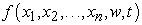.

The ESO dynamics are proposed as following equation: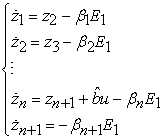(2)

In equation (2):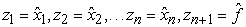(3)

State variable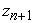is the estimate of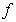and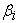are observer gains. Variable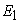is defined as follows: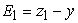(4)Coefficients are chosen so that the matrix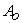is negative definite: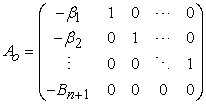(5)

For a second order system that stated by following equation we have: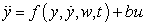(6)

Observer structure is: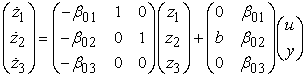(7)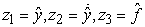(8)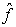is the estimation of the total disturbance. The control signal then can be built according to the following equation: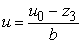(9)

Reduces (6) to a simple double-integral plant: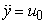(10)

This can be easily controlled. The difference between this and all of the previous methods is that no analytical model of 𝑓 is assumed here. The only thing required is the knowledge of the order of the plant model and the approximate value of b in (6) . For the control signal u0 is in the form of: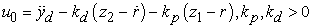(11)

Where r is the setpoint. The controller parameters,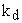and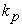, will be tuned by proposed methods in the next sections. Equation 11 shows that instead of using speed of output signal an estimate of it,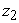, is used. So ADRC has no need to speed of the output signal and this is an advantage of ADRC algorithms.

It is proven in  ADRC is stable if the characteristic polynomial for the state feedback design (11)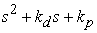is Hurwitz. So when the parameters ofandare positive ADRC is stable.

The gains observer: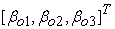(12)

To simplify the tuning process, we consider the parameters as: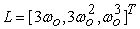(13)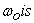observer bandwidth.

A number of studies have attempted to tune parameters of the ADRC [14, 15, 16, 17]. In this paper ARO and PSO, as two optimization methods, are used to determine the best value of b andandto achieve a proper response in a control problem. In the next section a proper objective function will be proposed.

2.2. Objective Function for Optimal ADRC

There are several limitations for overshoot and control signal. Therefore, in this paper integral of the squared derivative of error is added to The Integral of the Squared Error (ISE) to guarantee the smoothness of the response and the control signal and minimize the overshoot. So the proposed objective function is: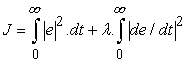(14)

Where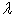is the smoothness weight coefficient of the response that varies between 0 and 1 and it is adjustable. If we need a smooth response without overshoot, we usenear 1. If we just focus on fast response and don’t mind about overshoot it should be near next sections two optimization methods are used to minimize this objective function.

2.3. Asexual Reproduction Optimization

Asexual Reproduction Optimization (ARO) is an individual and a model free optimization technique. It is proposed in 2010. ARO inspired from the budding procedure of asexual reproduction. ARO principally is an evolutionary algorithm. [19, 20]

Each individual produces a childthat in called bud. Then, they participate in a competition with each other and more deserving person is selected based on a performance directory that has been defined as an objective function optimization problem.

A binary string indicates each individual which is a decision variable vector, X = (x1, x2, . . .,xn); → X Rn. Each variable is characterized as the chromosome which prepared by a number of bits, called genes. Thus, for a chromosome with a length of L the first bit is the sign of the individual. The second and third bit of L shows the integer part, L1, and decimal part, L2, of the chromosome, respectively. So L = L1 + L2 + 1 and the length of each individual in the n-the dimensional space is Li = n × L [19, 20].

In ARO each solution in the search space is an organism in its environment that resources are limited and the most deserving organism can stay alive. To start the algorithm, an individual is accidentally initiated in the space S. The individual reproduces an offspring labeled bud by a particular operator known as reproduction mechanism. According to a performance index or a fitness function the parent and its offspring compete to stay alive. If the bud wins the competition, its parent will be rejected. Therefore, the bud is replaced with its parent and it becomes the new parent. If the parent wins the bud will be thrown away. When the stopping criteria are satisfied the algorithm is stopped. [19, 20].

2.4. Particle Swarm Optimization

Particle Swarm Optimization (PSO) algorithm was introduced for the first time in 1995. PSO is a population-based evolutionary optimization algorithm. It is inspired from social behavior of bird and fish. In this method, a swarm has a set of population members that called particle. A position and velocity are defined for each particle. The position indicates a nominee solution in the solution space. The velocity transfers it from one position to another in the multidimensional solution space. A fitness function is used to calculate the particle value of each position, until the convergence criteria are satisfied .

### 3. Case Study: Nonlinear Non-isothermal Continuous Stirred Tank Reactor, Implementation and Results

3.1. Case Study Descriptions

In this section, first ADRC that is tuned by ARO will be applied to a non-isothermal CSTR with constant parameters and its performance will be compared to ADRC tuned by PSO and both of them will be compared with PID control. Then, all of them will be applied to an exothermic CSTR with time varying parameters including activation energy and heat transfer coefficient.

Consider a simple liquid-phase, irreversible chemical reaction where chemical species A reacts to form species B. The reaction can be written as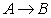. We assume that the rate of reaction is first-order with respect to component A: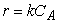(15)

Where r is the rate of reaction of A per unit volume, k is the reaction rate constant, and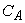is the molar concentration of species A. For single-phase reactions, the rate constant is typically a strong function of reaction temperature given by the Arrhenius relation: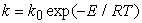(16)

Where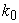is the frequency factor, R is the gas constant, and E is the activation energy.

The diagram of the CSTR is shown in Figure 2. The inlet flow consists of pure species A with molar concentration. Cooling coils, fix the reaction mixture at the desired operating temperature by removing heat that is produced in the reaction.

The process model consists of two nonlinear ordinary differential equations are given :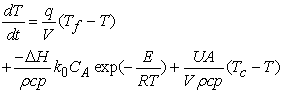(17)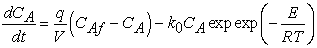(18)

Where TC, coolant temperature, is the manipulated variable; and T, reactor temperature, is the controlled variable. To produce a desire component concentration, coolant flow rate influences the reactor temperature and reaction rate. Here it is assumed that the coolant flow rate is fixed. Due to physical limitations and reactor safety it is necessary to control the temperature accurately. Other parameters are given in Table 1. 

The MATLAB software is employed for simulation and numerical solving the equations of the system’s dynamics described by Eq. (17), Eq. (18). Also, it is considered the continuous implementation with the sampling time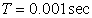. The values of equilibrium points are given in Table 1.

#### Table 1. Parameters of non-isothermal CSTR

3.2. Case Study 1: Temperature Control of Non-isothermal CSTR with Constant Parameters

The open-loop step responses to step changes in Tc is shown in Figure 3. It is seen that the process is strongly nonlinear and highly unstable.

In order to control the temperature of this oscillating plant, ADRC-, ADRC-PSO and PID approaches are applied. In Figure 4 the setpoint tracking behaviors of ADRC- and ADRC-PSO and PID are compared. For setpoint changes, as shown in Table 2, ADRC- shows a faster response than the ADRC-PSO control strategies. PID shows faster response than ADRC- but it delivers a very big overshoot and longer settling time in setpoint changes.

Figure 5 shows the disturbance rejection performance of ADRC-ARO and ADRC-PSO controller strategies. Because the performance of PID was so poor in disturbance rejection it is preferred not to compare it with other methods. For -30K change in inlet feed temperature at t=100, as disturbance, ADRC-ARO exhibits a faster response toward setpoint when compared to ADRC-PSO.

In comparison with ADRC-PSO and PID, ADRC-ARO shows better performance with much faster response to the setpoint.

Figure 3. Reactor temperature variation with step changes in cooling water temperature Tc from i) 300 K to 305 K and ii) 300 K to 290 K.
Figure 5. The disturbance rejection performance for -30 K step change in the inlet feed temperature (Tf) of the CSTR at t=100sec

#### Table 2. Set point tracking performance of controllers

3.3. Case Study 2: Temperature Control of the Non-isothermal CSTR with Time Varying Parameters

In this section, the previously ADRC-ARO and ADRC-PSO and PID control strategies will be applied to the non-isothermal CSTR with time varying parameters. Two case studies will be examined in which the CSTR shows strong nonlinearities. First, it is supposed that the heat transfer coefficient and so the overall heat transfer coefficient is varying with time due to fouling, then the Time varying activation energy caused by deactivation and regeneration of the catalyst are considered to show the robustness capability of ADRC approach.

3.3.1. Time Varying Heat Transfer Coefficient

Fouling is one of the most serious issues in heat transfer equipment. In the period of process operation, when a material is deposited on a heat transfer surface, Fouling happens. In practical applications, heat transfer surfaces usually become polluted with deposits and this makes extra resistance to the stream of heat. There are two usual cases fouling film over a period of time. One is asymptotic fouling. In this case, the resistances to heat transfer increases fast when the operation starts and becomes asymptotic to a steady state value at the end. The other one is linear fouling, where the fouling resistance increases linearly during the entire process operation. In this paper, we consider just second case that the fouling film increase linearly over the period of process operation. Therefore, the overall heat transfer coefficient is given by following equation :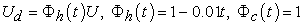(19)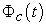and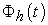are time-varying parameters which indicate the catalyst deactivation / regeneration and heat transfer fouling .

The open-loop step responses to step changes in Tc is shown in Figure 6. It is seen that the process is strongly nonlinear and non-stationary.

Figure 6. The open - loop response of the CSTR with the time varying heat transfer coefficient to step changes in cooling water temperature Tc from i) 300 K to 305 K and ii) 300 K to 290 K
Figure 8. The disturbance rejection performance for -30 K step change in the inlet feed temperature (Tf) of the CSTR with time-varying heat transfer coefficient at t=100sec

Figure 7 shows that the setpoint tracking performance of ADRC-ARO and ADRC-PSO are only slightly affected by the time varying heat transfer coefficient. PID controller, however, severely affected by fouling. In setpoint tracking ADRC-ARO has faster response with less settling time than ADRC- PSO. In disturbance rejection in Figure 8, ADRC-ARO exhibits faster response than ADRC-PSO. So ADRC-ARO for both setpoint tracking and disturbance rejection acts efficiently.

3.3.2. Time Varying Activation Energy

The temperature dependence of the rate expression is usually represented by the rate constant through the Arrhenius in (16).

Although the activation energy is not affected by temperature in the moderate temperature range, there are some exceptions. Other factors known to affect the activation energy include pressure and catalyst in the reaction. In the presence of a catalyst, an important factor which affects the rate of reaction is the deactivation of the catalyst by poisoning. There is no exact theoretical expression for the deactivation process. However, some empirical expressions have been reported. In this case study, the general equation for k is given by :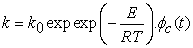(20)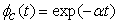(21)

3.3.2.1. Catalyst Deactivation (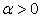)

In the presence of a catalyst, the reaction rate is greatly influenced by the catalyst condition. During the period of reaction, because of poisoning, the catalyst may be deactivated. The catalyst deactivation is usually unpredictable, so an empirical correlation is used just for the simulation purpose.

Figure 9. The open-loop response of the CSTR with time varying activation energy due to catalyst deactivation coefficient to step changes in cooling water temperature Tc from i) 300 K to 305 K and ii) 300 K to 290 K.

The open-loop responses to step changes in Tc is shown in Figure 9.

The setpoint tracking and disturbance rejection of different controllers are shown in Figure 10 and Figure 11. We can see that the performance of the ADRC-ARO is much better than ADRC-PSO and PID in setpoint tracking. In Figure 11 the temperature of the inlet feed (Tf) is decreased by -30k and shows that both ADRC-ARO and ADRC-PSO methods reject it in less than 30 second. Obviously the performance of ADRC-ARO in setpoint tracking and disturbance rejection is better than ADRC-PSO.

Figure 10. Setpoint tracking performance of CSTR with time varying activation energy due to catalyst deactivation ()
Figure 11. The disturbance rejection performance for -30 K step change in the inlet feed temperature (Tf) of the CSTR with time-varying activation energy due to catalyst deactivation at t=100sec.

3.3.2.2. Catalyst Regeneration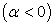Catalyst regeneration is a last case that is considered in this work. During this process, the catalyst is reactivated and the reaction rate can get to be very large. Catalyst regeneration could be an extremely serious situation. A great amount of heat is produced; however, it cannot be removed in time. This may result in the reactor to lose temperature control and equipment damage. Once in a while a blast may cause extreme harm. The open-loop simulation shown in Figure 12 exhibits this point.

Figure 12. The open-loop response of the CSTR with time varying activation energy due to catalyst regeneration to step changes in cooling water temperature Tc from i) 300 K to 305 K and ii) 300 K to 290 K.

In Figure 13 and Figure 14 the performance of controllers in setpoint tracking and disturbance rejection are demonstrated. Figure 13 shows that the performance of the ADRC-ARO is much better than ADRC-PSO and ADRC-PSO is better than PID because it has less settling time and overshoot. In Figure 13 Similar to constant parameter and time varying heat transfer coefficient, PID method starts with a big overshoot and is affected more from regeneration. So unlike ADRC-ARO and ADRC-PSO, performance of PID is seriously influenced by this change which is so dangerous and can damage to the reactor. Overall the performance of ADRC-ARO is more superior than other controllers mentioned in this paper.

Figure 14. The disturbance rejection performance for -30K change in the inlet feed temperature (Tf) of the CSTR with time-varying activation energy due to catalyst regeneration at t=100sec.

### 4. Conclusion

In this paper, an optimal active disturbance rejection controller, ADRC-, was proposed to control the temperature of the . The ADRC-ARO is applied to a non-isothermal CSTR. First, the performance of this approach was studied in CSTR with constant parameters and then with time varying parameters including heat transfer coefficient due to fouling and activation energy caused by deactivation and regeneration of the catalyst. The performance of ADRC- was compared with ADRC-PSO approach. ADRC-ARO revealed Improvement specially in setpoint tracking with faster response time and less settling time in all case studies in this paper. Then both approaches have been compared with PID control. Obviously, ADRC-ARO and ADRC-PSO performed better than PID, this comparison applied just because we have seen the extreme scope of the nonlinearity by PID control. Finally, it has been proven that ADRC-ARO has an excellent performance in controlling the non-isothermal CSTR in the situation when the PID controller failed. It has been demonstrated that the ADRC-ARO controller is robust enough in an extensive range of operating conditions. Generally, if the parameters of ADRC tuned properly, it performs more efficiently in setpoint tracking and rejecting external and un-modeled disturbances.

### Acknowledgement

The authors would like to thank the technical and financial supports provided by the National Iranian Gas Company–Sarkhoon & Qeshm Gas treating Company (NIGC-SQGC).

### References

  A. Favache and D. Dochain, “Power-shaping control of reaction systems: The CSTR case,” Automatica, vol. 46, pp. 1877-1883, 2010.In article CrossRef  F. Martinsen, L. T. Biegler, and B. A. Foss, “A new optimization algorithm with application to nonlinear MPC,” Journal of Process Control, vol. 14, pp. 853-865, 2004.In article CrossRef  C.-T. Chen and S.-T. Peng, “Intelligent process control using neural fuzzy techniques,” Journal of process control, vol. 9, pp. 493-503, 1999.In article CrossRef  W.-D. Chang, “Nonlinear CSTR control system design using an artificial bee colony algorithm,” Simulation Modelling Practice and Theory, vol. 31, pp. 1-9, 2013.In article CrossRef  V. Ghaffari, S. V. Naghavi, and A. Safavi, “Robust model predictive control of a class of uncertain nonlinear systems with application to typical CSTR problems,” Journal of Process Control, vol. 23, pp. 493-499, 2013.In article CrossRef  D. E. Seborg, D. A. Mellichamp, T. F. Edgar, and F. J. Doyle III, Process dynamics and control: John Wiley & Sons, 2010.In article  H. Jingqing, “Auto-disturbances-rejection Controller and Its Applications [J],” Control and Decision, vol. 1, 1998.In article  J. Han, “From PID to active disturbance rejection control,” Industrial Electronics, IEEE transactions on, vol. 56, pp. 900-906, 2009.In article  J. Han, “Active disturbance rejection control technique-the technique for estimating and compensating the uncertainties,” National Defense Industry Press, Beijing, pp. 197-270, 2008.In article PubMed  B. Sun and Z. Gao, “A DSP-based active disturbance rejection control design for a 1-kW H-bridge DC-DC power converter,” Industrial Electronics, IEEE Transactions on, vol. 52, pp. 1271-1277, 2005.In article CrossRef  D. Wu, X. Wang, T. Zhao, and W. Lv, “Application of active disturbance rejection to tracking control of a fast tool servo system,” in Control Applications, 2005. CCA 2005. Proceedings of 2005 IEEE Conference on, 2005, pp. 547-552.In article  Q. Zheng and Z. Gao, “An energy saving, factory-validated disturbance decoupling control design for extrusion processes,” in Intelligent Control and Automation (WCICA), 2012 10th World Congress on, 2012, pp. 2891-2896.In article  C.-E. Huang, D. Li, and Y. Xue, “Active disturbance rejection control for the ALSTOM gasifier benchmark problem,” Control Engineering Practice, vol. 21, pp. 556-564, 2013.In article CrossRef  H.-S. Li and X.-F. Zhu, “On parameters tuning and optimization of active disturbance rejection controller,” Control Engineering of China, vol. 11, pp. 419-423, 2004.In article  Y.-l. SHI, C.-z. HOU, and H.-b. SU, “Auto-disturbance-rejection controller design based on particle swarm optimization algorithm,” Journal of System Simulation, vol. 2, p. 040, 2008.In article  Z.-j. KANG, Q.-w. WANG, L. Huang, B. LIU, and L. XU, “Design of Auto-disturbance-rejection Controller of Rectifier in HVDC System Based on the Improved Genetic Algorithm [J],” High Voltage Engineering, vol. 8, p. 017, 2008.In article  Z. Gao, “Scaling and bandwidth-parameterization based controller tuning,” in Proceedings of the American Control Conference, 2006, pp. 4989-4996.In article  Z. Gao, “Active disturbance rejection control: a paradigm shift in feedback control system design,” in American Control Conference, 2006, 2006, p. 7 pp.In article  T. Mansouri, A. Farasat, M. B. Menhaj, and M. Reza Sadeghi Moghadam, “ARO: A new model free optimization algorithm for real time applications inspired by the asexual reproduction,” Expert Systems with Applications, vol. 38, pp. 4866-4874, 2011.In article CrossRef  A. Farasat, M. B. Menhaj, T. Mansouri, and M. R. S. Moghadam, “ARO: A new model-free optimization algorithm inspired from asexual reproduction,” Applied Soft Computing, vol. 10, pp. 1284-1292, 2010.In article CrossRef  Y. Shi and R. Eberhart, “A modified particle swarm optimizer,” in Evolutionary Computation Proceedings, 1998. IEEE World Congress on Computational Intelligence., The 1998 IEEE International Conference on, 1998, pp. 69-73.In article  J. B. Rawlings and J. G. Ekerdt, Chemical reactor analysis and design fundamentals: Nob Hill Pub, Llc, 2002.In article  R. Perry and C. Chilton, “1973, Chemical Engineers Handbook,” ed: McGraw–Hill, New York.In article